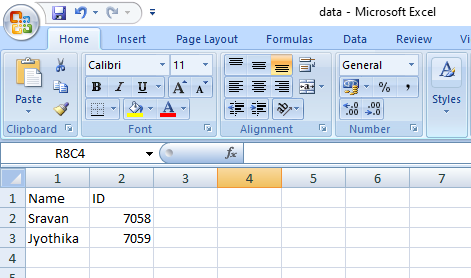GeeksforGeeks App
Open AppBrowser
Continue

# How to create matrix and vector from CSV file in R ?

In this article, we will discuss how to convert CSV data into a matrix and a vector in R Programming Language. We will use read.csv() function to load the csv file:

where, path is the location of a file present in our local system.

Matrix: Matrix is a two-dimensional data structure that contains rows and columns. It can hold multiple data types. We can convert csv file data into matrix by using the method called as.matrix()

Syntax:as.matrix(csv_file_object)

Vector: Vector is a one-dimensional data structure that can hold multiple datatypes. We can convert CSV data into a vector, By using as.vector()

Syntax: as.vector(csv_file_object)

CSV File Used:Step 1: Create an object to CSV by reading the path

## R

 `data=``read.csv``(``"C:/sravan/data.csv"``)``print``(data)`

Output:

```    Name       ID
1  sravan    7058
2  Jyothika  7059```

Step 2: Convert the data into a matrix.

## R

 `matrixdata = ``as.matrix``(data)``print``(matrixdata)`

Output:

```    Name           ID
[1, ] "sravan"   "7058"
[2, ] " Jyothika" "7059"```

Step 3: Convert the data into a vector

## R

 `vectordata=``as.vector``(data)``print``(vectordata)`

Output:

```    Name       ID
1  sravan    7058
2  Jyothika  7059```

Below is the full implementation:

## R

 `# Read data from CSV``data=``read.csv``(``"C:/sravan/data.csv"``)`` ` `# Create a matrix``matrixdata=``as.matrix``(data)`` ` `# Create a vector``vectordata=``as.vector``(data)``print``(matrixdata)``print``(vectordata)`

Output:

```    Name           ID
[1, ] "sravan"   "7058"
[2, ] " Jyothika" "7059"
Name       ID
1  sravan    7058
2  Jyothika  7059```

My Personal Notes arrow_drop_up